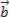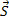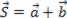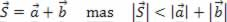# Sum of vectors

Traffic sign representing two-way traffic through the use of two vectors

As we already know, a vector is a mathematical entity that represents a set of oriented line segments, whose function is to provide module, direction and direction information. Let’s consider any two non-zero vectorsand(figure below).

From the end, we draw a vector equal to. Connecting the origin of the first vector with the end of the second vector, we obtain the vector, which is called the sum vector or vector resulting from a and b :.

We can also proceed as indicated in the figure below, that is, from the end ofwe draw a vector equal to. The result we will get is the same as the previous one:It is important to note that in this case the length ofis not equal to the sum of the lengths ofand: